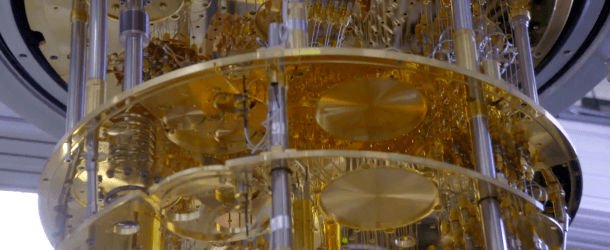(TechRepublic) Measuring the computational ability of quantum computers is a complex problem. Counting the number of qubits in a quantum computer to determine computational power is too simplistic to be functionally useful.
A standard for measuring the computational ability of quantum computers was proposed by IBM in 2017, called “quantum volume.” Quantum volume is measured by calculating the number of physical qubits, connectivity between qubits, and time to decoherence, as well as the available hardware gate set, and number of operations that can be run in parallel.
According to the researchers who defined quantum volume, that metric “enables the comparison of hardware with widely different performance characteristics and quantifies the complexity of algorithms that can be run.” Likewise, the researchers noted that quantum volume can only increase if the number of qubits, and the error rate of those qubits, increase in parallel.